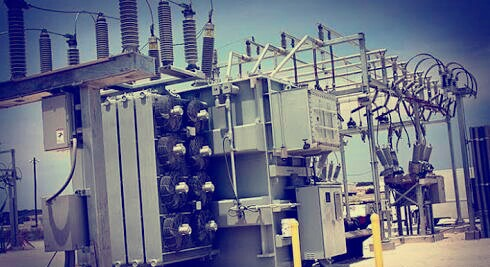# All Day Efficiency of Transformer/ Distribution Transformer All Day Efficiency

In our previous articles we have discussed transformer working, construction etc.Today we discuss one of the important parameter of distribution transformer I.e, “all day efficiency of a distribution transformer”.We know for a transformer, the efficiency is defined as the ratio of output power to input power.

Transformer Efficiency= Output Power /Input Power

The above equation is efficiency of any transformer. But for some special types of transformers such as distribution transformers power efficiency is not the true measure of the performance.For that purpose distribution transformer we calculate all day efficiency.Distribution transformer serve residential and commercial loads.The load on distribution transformers vary considerably during the period of the day. For most period of the day these transformers are working at 30 to 40 % of full load only or even less than that. But the primary of such transformers is energised at its rated voltage for 24 hours, to provide continuous supply to the consumer.
The core loss which depends on voltage, takes place continuously for all the loads. But copper loss depends on the load condition. For no load, copper loss is negligibly small while on full load it is at its rated value. Hence power efficiency can not give the measure of true efficiency of distribution transformers. in such transformers, the energy output is calculated in kilo watt hour (kWh). Then ratio of total energy output to total energy input (output + losses) is calculated. Such ratio is called energy efficiency or All Day Efficiency of a transformer.
Based on this efficiency, the performance of various distribution transformers is compared. All day efficiency is defined as,While calculating energies, all energies can be expressed in watt hour (Wh) instead of kilo watt hour (kWh). Such distribution transformers are designed to have very low core losses. This is achieved by limiting the core flux density to lower value by using a relative higher core cross-section i.e. larger iron to copper weight ratio.
The maximum efficiency in distribution transformers occurs at about 60-70 % of the full load. So by proper designing, high energy efficiencies can be achieved for distribution transformers. Numerical problems on all day efficiency of a transformer will be posted soon.